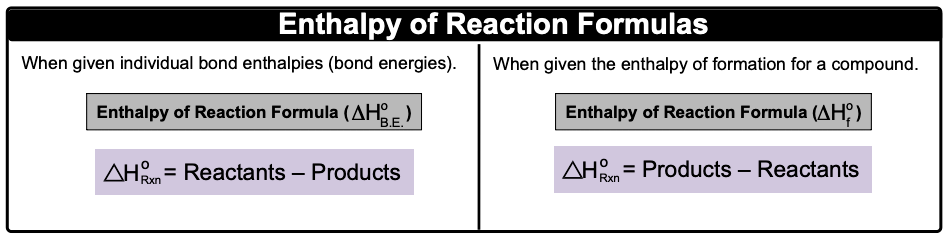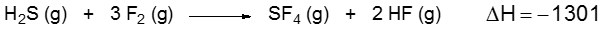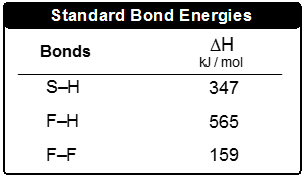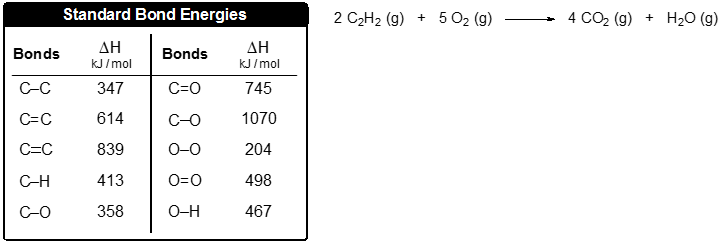Clutch Prep is now a part of Pearson
Ch.9 - Bonding & Molecular StructureWorksheetSee all chapters

# Bond Energy

See all sections
Sections
Lewis Dot Symbols
Chemical Bonds
Dipole Moment
Octet Rule
Formal Charge
Lewis Dot Structures: Neutral Compounds
Lewis Dot Structures: Sigma & Pi Bonds
Lewis Dot Structures: Ions
Lewis Dot Structures: Exceptions
Lewis Dot Structures: Acids
Resonance Structures
Average Bond Order
Bond Energy
Coulomb's Law
Lattice Energy
Born Haber Cycle

Bond Energy is the amount of energy stored within a chemical bond.

###### Bond Energy

Concept #1: Bond Energy

When individual bond energies are given, we will use the first formula.Example #1: Calculate the ∆Horxn if the bond enthalpies of NΞN, H–H and N–H are 945 kJ/mol, 432 kJ/mol and 391 kJ/mol respectively.

Practice: Consider the following equation:Determine the bond enthalpy value for the F–S bond.Practice: Use the bond energies to estimate the enthalpy of reaction for the combustion of 5 moles of acetylene: